## Python: Usage of Triple Quotes (“””)It is quite rare for myself to have chance to read people Python’s code because none of my circle of friends are coding in Python. So I am not able to conclude how often people used the triple quotes (“””). Python allows single quotes and double quotes as well as triple quotes. While single and double quotes work similarly, triple quotes do something special.

The below is the extraction from my first Python’s blog entry.

We can use triple quotes (“””) for a string to span multiple lines and assign it to a variable. One of the examples I learned,

haiku = “””The old pond,
A frog jumps in:
Plop!, we expected: The old pond,
A frog jumps in:
Plop!”””

Another usage of triple quotes (“””) as docstrings. Docstrings describe what the function does. It serves as a documentation for the function and it is placed immediately after the function’s header.

```def square (value):
"""Return a value of the square"""
new_value = value ** 2
return(new_value)
```

Next time, when seeing triple quotes, you will know it serves as a documentation too within a function.

## Day 33: Transpose a Numpy Array in PythonIn the last few exercises in the Intermediate Python module in DataCamp, I learned how to do transpose on a Numpy array in Python. The code is simple and it handles by the Numpy package without hassle. Let us look into the code snippet below:

```# numpy and matplotlib imported, seed set.

# initialize and populate all_walks
all_walks = []
for i in range(10) :
random_walk = 
for x in range(100) :
step = random_walk[-1]
dice = np.random.randint(1,7)
if dice <= 2:
step = max(0, step - 1)
elif dice <= 5:
step = step + 1
else:
step = step + np.random.randint(1,7)
random_walk.append(step)
all_walks.append(random_walk)

# Convert all_walks to Numpy array: np_aw
np_aw = np.array(all_walks)

# Plot np_aw and show
plt.plot(np_aw)
plt.show()

# Clear the figure
plt.clf()

# Transpose np_aw: np_aw_t
np_aw_t = np.transpose(np_aw)

# Plot np_aw_t and show
plt.plot(np_aw_t)
plt.show()
```

Before the transpose takes place at the line of codes,
np_aw_t = np.transpose(np_aw)

the line plot which I plotted looks as below: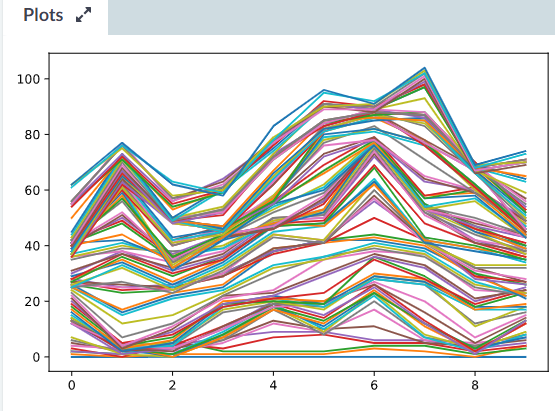When I print out the np_aw array, the output looks as below:

[[ 0 0 1 … 52 51 50]
[ 0 1 2 … 60 59 60]
[ 0 0 1 … 87 88 89]

[ 0 1 2 … 69 70 71]
[ 0 1 0 … 99 98 99]
[ 0 2 1 … 61 60 61]]

It is like of mess and I did not know what is what…

Then, the next line of codes, transpose the np_aw array. It looks as below when I print out the np_aw_t array.

[[ 0 0 0 … 0 0 0]
[ 0 1 0 … 1 1 2]
[ 1 2 1 … 2 0 1]

[52 60 87 … 69 99 61]
[51 59 88 … 70 98 60]
[50 60 89 … 71 99 61]]

And, the line plot looks as below: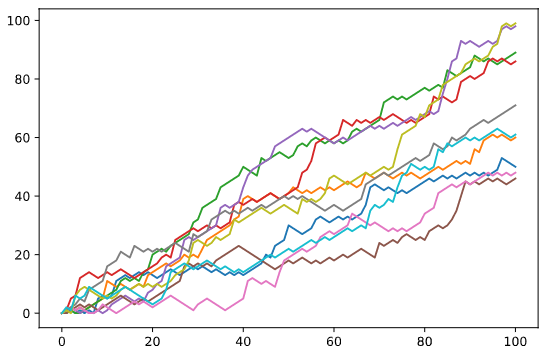## Day 32: Visualization with Random NumbersIn one of my DataCamp’s exercise, it combines the randint() function from the Numpy package, the built-in max() function and matplotlib.pyplot package to do a simple visualization of random steps (random_walk) over 100 times with if-elif-else condition to increase or decrease the step’s value for each iteration. Then, it appends into the random_walk list to generate the line plot.

```# Numpy is imported, seed is set

# Initialization
random_walk = 

for x in range(100) :
step = random_walk[-1]
dice = np.random.randint(1,7)

if dice <= 2:
step = max(0, step - 1)
elif dice <= 5:
step = step + 1
else:
step = step + np.random.randint(1,7)

random_walk.append(step)

# Import matplotlib.pyplot as plt
import matplotlib.pyplot as plt

# Plot random_walk
plt.plot(random_walk)

# Show the plot
plt.show()
```

If you notice, the plt.plot takes only one argument. Usually, the plot() requires to have two values, (x,y) for the x-axis and y-axis. Python knows what to do when only one argument passes to plot().

In this scenario, it uses the index of the list to map onto the x-axis, and the values in the list onto the y-axis.

So, the generated line plot looks as below: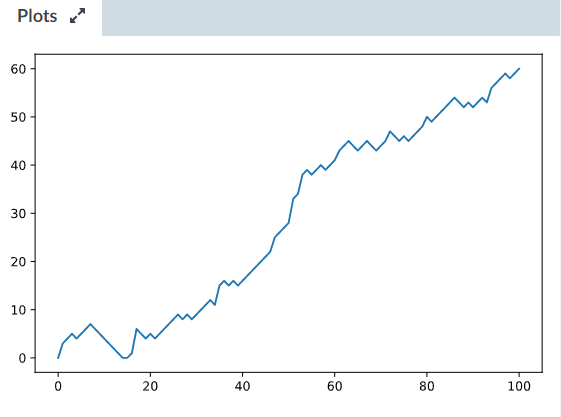## Day 32: Random Number in PythonIn Python, we can use the random module to generate pseudo-random numbers ranging between zero and one.

Example:

```from random import *

print random()     # Generate a pseudo-random number between 0 and 1.
```

Random number between 1 and 100
To generate a whole number (integer) between one and one hundred, example:

```from random import *

print randint(1, 100)    # Pick a random number between 1 and 100.
```

Of course, you can also store the value in a variable instead of print out.

Random number between 1 and 10
To generate a random floating point number between 1 and 10, we can use the uniform() function.

```from random import *

print uniform(1, 10)
```

Random Generators using Numpy
It uses the rand() function, example as below:

```# Import numpy
import numpy as np

# Random rand()
np.random.rand()
```

It returns values between 0 and 1, similar to the random() function above.

We can set a seed number where the same seed number will generate the same random number. This is useful because it ensures ‘reproducibility’ where it can be applied into running different versions of the same algorithm and you are using the exact same random numbers and making a fair comparison between the versions.

```# Import numpy
import numpy as np

# Random rand() with seed
np.random.seed(10)
np.random.rand()

# This is different because we did not use seed
np.random.rand()
```

Using the randint()
WIth Numpy randint(), we can specify the range of whole number to be randomly generated. The arguments for randint(),

numpy.random.randint(low, high=None, size=None, dtype=’l’)
low: smallest signed number
high: optional. Largest signed number, but excluded from the random.
size: optional. Output shape.
dtype: optional. Desired dtype of the result.

```# Import numpy
import numpy as np

# Random rand() with seed
np.random.seed(10)
coin = np.random.randint(0,2)
print(coin)
```

Since the above code uses the seed number, the output will be same for each time we run the codes.

In the exercise given by DataCamp, it requires,
1. Roll the dice using randint() and assign to a variable, dice.
2. Use if-elif-else condition to construct below:

If dice is 1 or 2, you go one step down.
If dice is 3, 4 or 5, you go one step up.
Else, you throw the dice again. The number of eyes is the number of steps you go up.

3. Print out dice and step and check if the output is correctly done.

```# Numpy is imported, seed is set

# Starting step
step = 50

# Roll the dice
dice = np.random.randint(1,7)

# Finish the control construct
if dice <= 2 :
step = step - 1
elif dice >= 3 and dice <=5 :
step = step + 1
else :
step = step + np.random.randint(1,7)

# Print out dice and step
print(dice)
print(step)
```

## Day 31: Looping in Pandas DataFrameI have covered the introduction of the Pandas and filtering in the Pandas DataFrame. Also, I covered the looping in the Numpy array. In this entry, I continue sharing about looping, this time is looping in Pandas DataFrame.

In the example given by DataCamp, it reused the existing brics.csv file to explain. Let me include the screenshot of the DataFrame.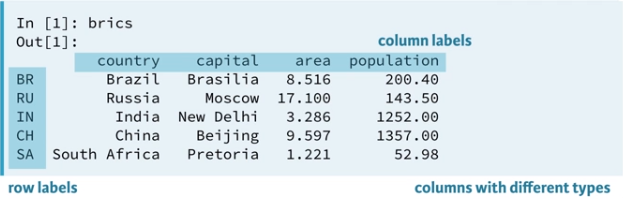When we use a for loop to loop through the brics and print out the value, the output seems not what we want to see. Let see the example below:

```# Import pandas as pd
import pandas as pd
# Import the brics
brics = pd.read_csv('brics.csv', index_col = 0)
# Use For loop
for val in brics:
print(val)
```

The output is showing the header of the DataFrame, just like below: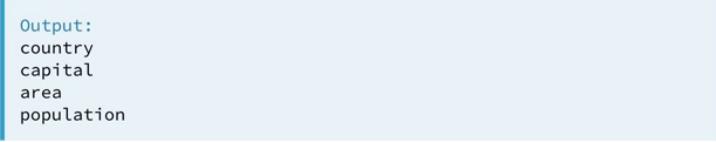This is not what we want to do by using for loop.

iterrows()
It iterates over the DataFrame rows as (index, series) pairs.

The syntax:
DataFrame.iterrows()

Let’s look into the example shared.

```# Import pandas as pd
import pandas as pd
# Import the brics
brics = pd.read_csv('brics.csv', index_col = 0)
# Use For loop
for lab, row in brics.iterrows():
print(lab)
print(row)
```

The output of the above codes shows a few different set of data by countries return to the screen. The first iteration returns ‘BR’ and the entire rows are Pandas series, follows by ‘RU’ and etc.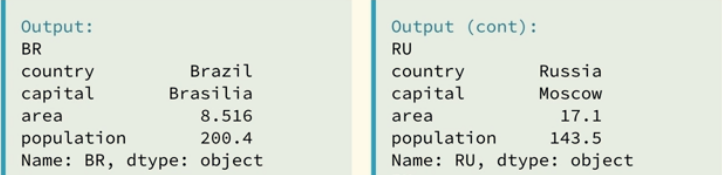Selective Print
If we want to print out the capital column’s values only for each iteration, refer to below codes:

```# Import pandas as pd
import pandas as pd
# Import the brics
brics = pd.read_csv('brics.csv', index_col = 0)
# Use For loop
for lab, row in brics.iterrows():
print(lab + ": " + row["capital"])
```

The output of the above code is expected to be: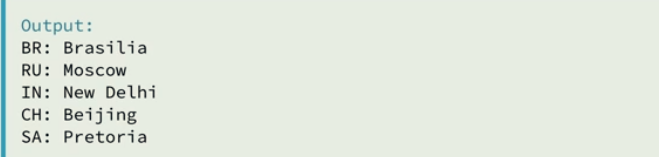By using the pandas.DataFrame.apply, it applies function along the input axis of DataFrame.

```# Import pandas as pd
import pandas as pd
# Import the brics
brics = pd.read_csv('brics.csv', index_col = 0)

brics["name_length"] = brics["country"].apply(len)
print(brics)
```

The output of the print(brics) shows a new column, “name_length” is added into the DataFrame and the value is the length of the country’s name in the country column. See below for the captured screenshot: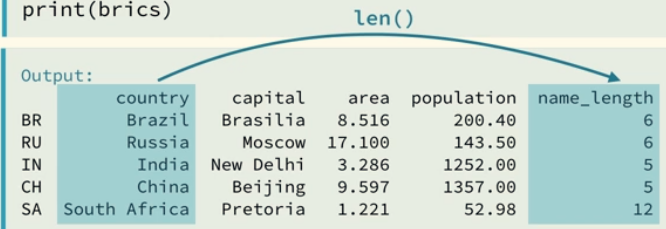In my exercise. I need to use a for loop to add a new column, named ‘COUNTRY’ that contains an uppercase version of the country names in the column ‘country’. My codes and the output of the execution as below:

```# Import cars data
import pandas as pd
cars = pd.read_csv('cars.csv', index_col = 0)

# Code for loop that adds COUNTRY column
for lab, row in cars.iterrows():
cars.loc[lab, "COUNTRY"] = row['country'].upper()

# Print cars
print(cars)
```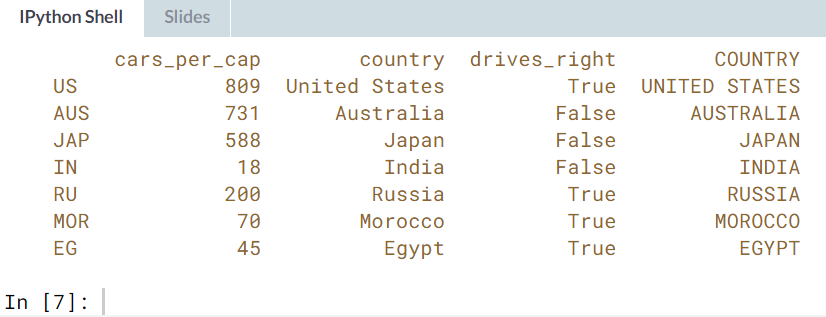And this can be achieved using 1 line of code and the Pandas.DataFrame.apply function, similar to the example above.

```# Import cars data
import pandas as pd
cars = pd.read_csv('cars.csv', index_col = 0)

# Use .apply(str.upper)
cars["COUNTRY"] = cars["country"].apply(str.upper)
print(cars)
```

## Aston SpecialitiesIt has been long time I did not visited Aston. I think the last vist was somewhere in late November last year to celebrate my aunt’s birthday at the Suntec City. This time I visited the branch at City Square, near Farrer Park MRT station.

The queue on Friday’s lunch on the day I visited the branch was quite long and it was quite crowded with working people, families and students as well.

It took us a while to come to our turn to place order at the counter before they lead us to our table. While waiting, I was unable to decide whether I want to eat the salmon with 2 side dishes or spaghetti with salmon. Then, I saw their new promotion, the chicken confit set which comes with 2 side dishes too. However, when it came to my turn to place order, the chicken confit was sold for the day. Wow!

So, I settled down with just the basic char-grilled chicken with garden veggie and potato wedges as my side dishes.The char-grilled chicken completes with mushroom sauce on top of it makes my lunch so completed. The chicken chop is slightly over-grilled where I saw some burned bites. However, other part of the chicken meat is still tender and juicy.

The poached garden veggie is completely over-cooked until it is too soft for me. Probably, this is suitable for people who prefer to eat soft broccoli and carrot.

Lucky the potato wedges saved my day, it is definitely cannot go wrong with processed food.

## Deliveroo: Street of Saigon

Rice vermicelli with grilled chicken and deep fried spring rollsIt was nicely presented in the takeaway box by the Deliveroo’s staff when my colleagues and myself dine-in the Deliveroo’s Kitchen at CT HUB 2, Lavender, Singapore. It comes with some chilies mixed fish sauce in a small container.

The amount of grilled chicken is very generous, definitely enough for you to go with the rice vermicelli and the meat is well cooked, just it looks a little bit dry and it is not oily.

The deep fried spring rolls are disappointed, why? When it means deep fried, I expected it to be less oily if the cook knows how to deep fried food. Maybe, deep fried spring rolls are different than other deep fried food? But hey, the amount of yellowish oils dripped down on to my plastic spoon is not healthy at all and it looks pretty disgusting. I have to use the table tissues to absorb all the excessive oils, or you may want to say (sauce!). I’m sure, not going to order this anymore. The deep fried spring rolls need improvement, or just serve it fresh.